# JAVA Why Java Java was design for commercial

• Slides: 62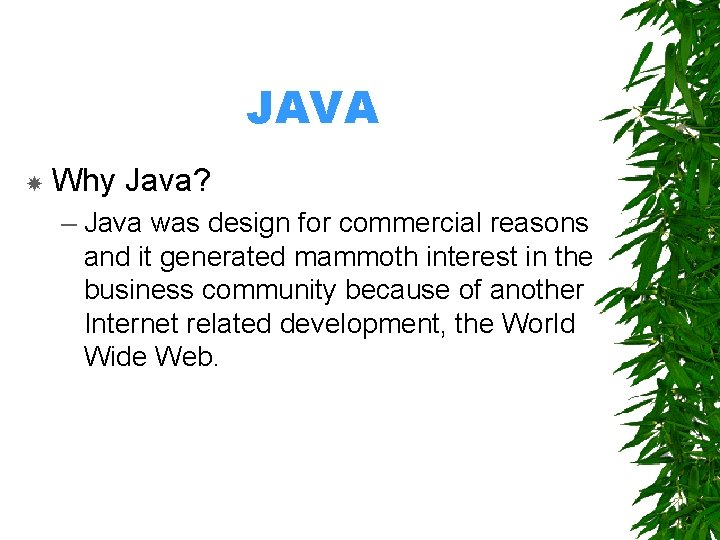JAVA Why Java? – Java was design for commercial reasons and it generated mammoth interest in the business community because of another Internet related development, the World Wide Web.Rule for Java Language • • C a s e n s i t i v e Class name and file name must be the same Class name can not have space E x t e n s i o n m u s t b e. j a v aCommon Error in Java • Omitting Semicolons • Misspelling wordsThere are two common Error in Java A p p l i c a t i o n Run as a stand alone program with java run-time E x. H e l l o. j a v a a s y o u t r y A p p l e t Run with java-enabled browser (IE, Netscape, etc. )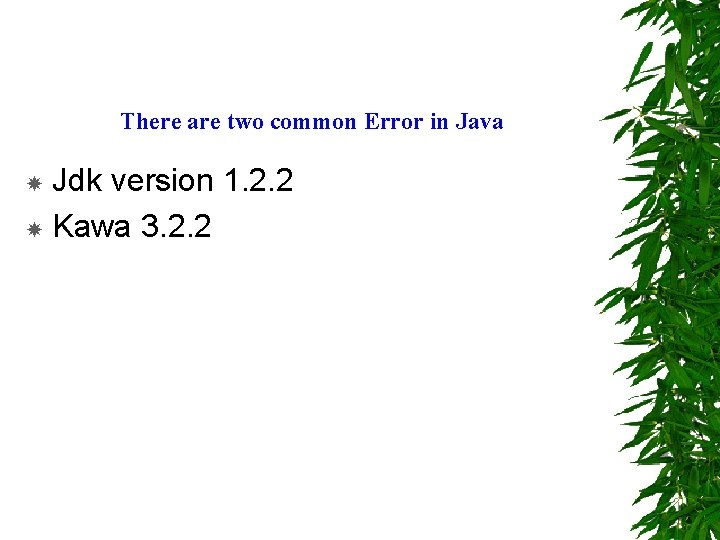There are two common Error in Java Jdk version 1. 2. 2 Kawa 3. 2. 2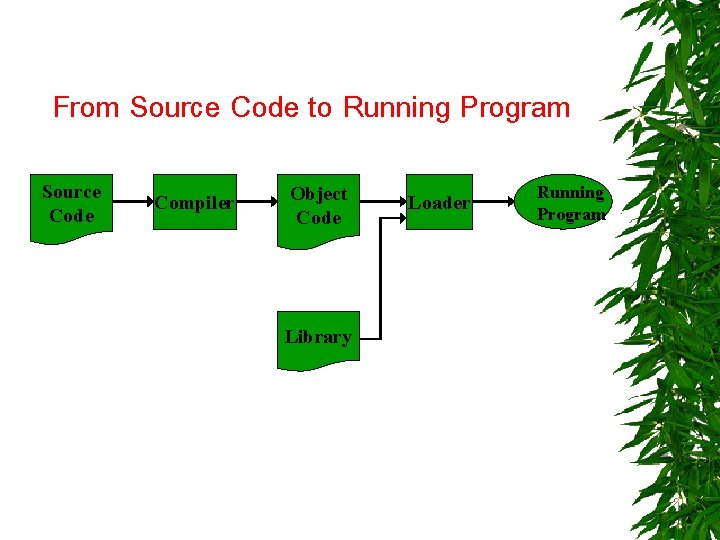From Source Code to Running Program Source Code Compiler Object Code Library Loader Running Program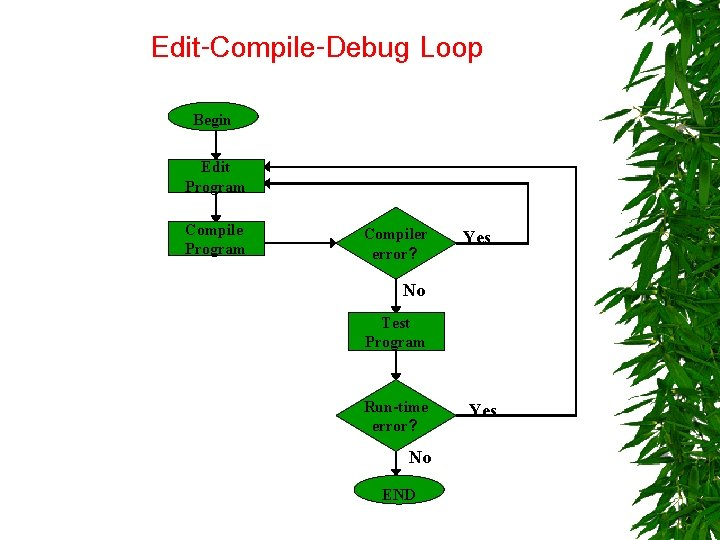Edit-Compile-Debug Loop Begin Edit Program Compiler error? Yes No Test Program Run-time error? No END Yes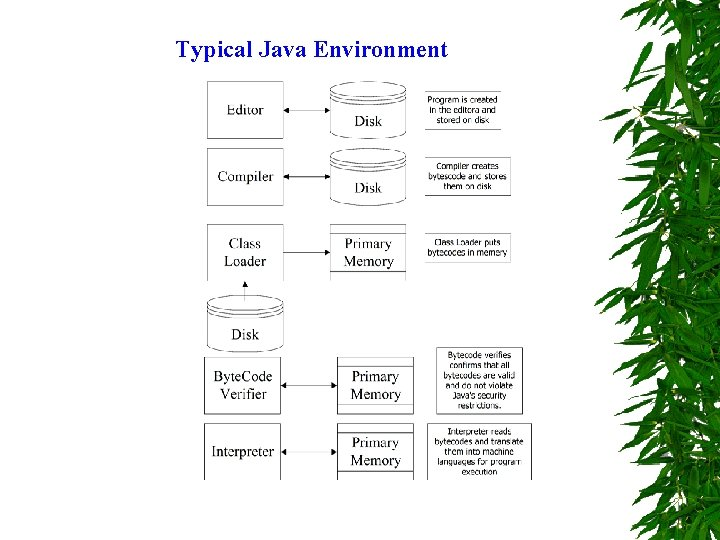Typical Java EnvironmentIntroduction to Java Programming Hello. java //A first Java Program import java. applet. Applet; import java. awt. Graphics; public class Hello extends Applet { public void paint (Graphics g) { g. draw. String ("Hello World", 25); } }HTML file <html> <applet code="Hello. class" width=275 height=135> </applet> </html>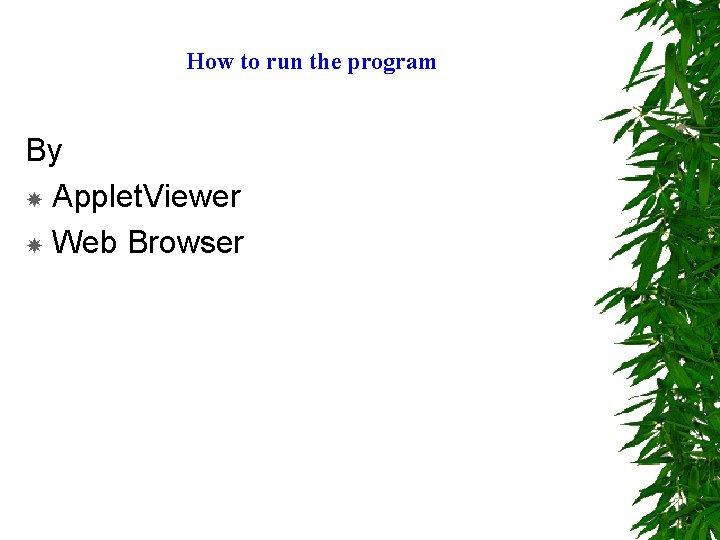How to run the program By Applet. Viewer Web Browser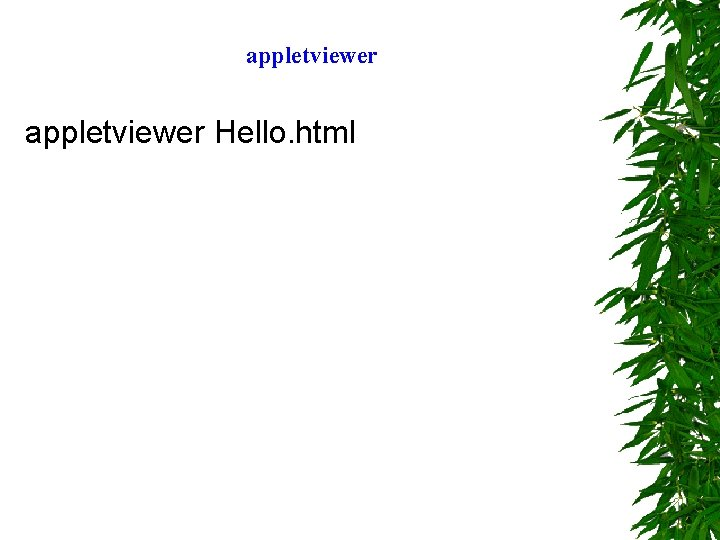appletviewer Hello. htmlWeb BrowserHello 2. java //Second Java Program import java. applet. Applet; import java. awt. Graphics; public class Hello 2 extends Applet { public void paint (Graphics g) { g. draw. String ("Hello World", 25); g. draw. String ("How are You? ", 25, 40); } }Addition. java //Another Java Program import java. applet. Applet; import java. awt. *; public class Addition extends Applet { Label prompt; Text. Field input; int number; int sum; public boolean action (Event e, Object o) { number=Integer. parse. Int(o. to. String()); input. set. Text(""); sum=sum+number; show. Status(Integer. to. String(sum)); return true; } } public void init() { prompt = new Label("Enter interger and Press Enter"); input = new Text. Field(10); add(prompt); add(input); sum=0; }Ranges of Data Types Type Size in bits boolean 1 char 16 byte 8 short 16 int 32 long 64 float double 32 64 Values true or false ‘u 0000’ to ‘u. FFFF’ -128 to +127 -32, 768 to +32, 767 -2, 147, 483, 648 to +2, 147, 483, 647 -9, 223, 372, 036, 854, 775, 808 to +9, 223, 372, 036, 854, 775, 807 -3. 40292347 E+38 to 3. 40292347 E+38 +1. 79769313486231570 E+308 to 1. 79769313486231570 E+308Constant With final keyword: public static final double PIE = 3. 1428; /*constant value for PIE */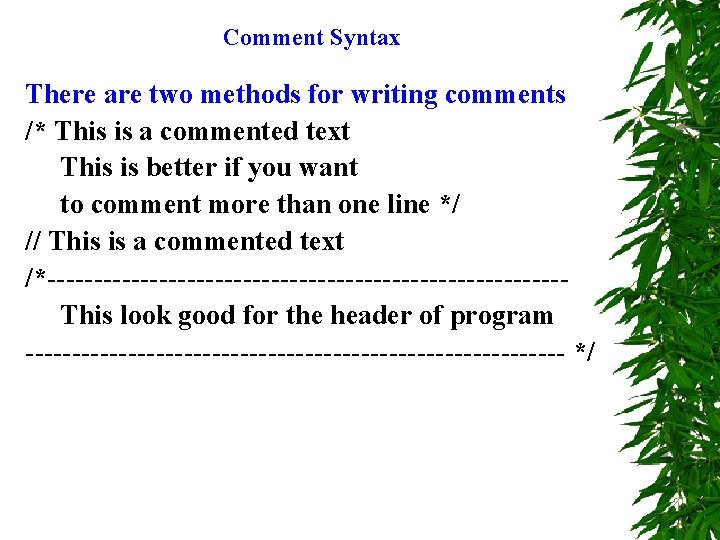Comment Syntax There are two methods for writing comments /* This is a commented text This is better if you want to comment more than one line */ // This is a commented text /*----------------------------This look good for the header of program ----------------------------- */Increment and Decrement Assignment Sample Assings operator Explanation Expression Assume: int c = 3, d = 5, e = 4, f = 6, g = 12; += -= *= =/ =% c += 7 d -= 4 e *= 5 f /= 3 g %= 9 c=c+7 d=d-4 e=e*5 f=f/3 g=g%9 10 to c 1 to d 20 to e 2 to f 3 to gIncrement and Decrement Operator Called Sample expression Explanation Increment a by 1 then use the new value of a in the expression in which a resides. Use the current value of a in the expression in which a resides , then increment a by 1. Decrement b by 1 then use the new value of b in the expression in which b resides. Use the current value of b in the expression in which b resides , then Decrement b by 1. ++ preincrement ++a ++ postincrement a++ -- predecrement --b -- postdecrement b--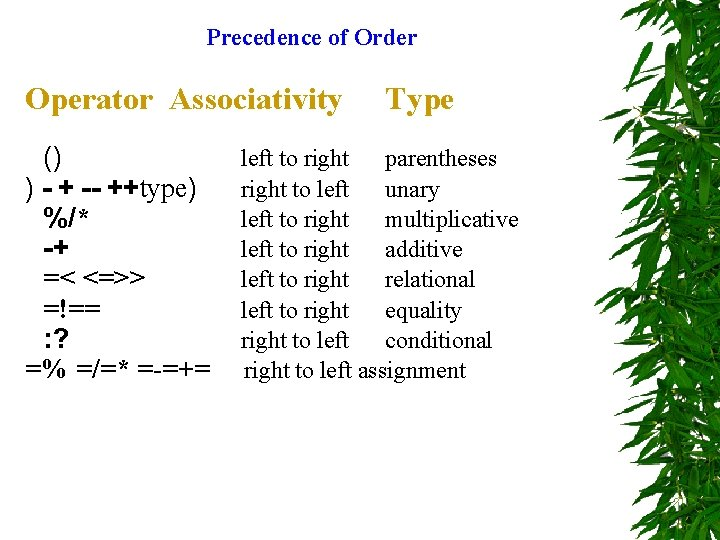Precedence of Order Operator Associativity () ) - + -- ++type) %/* -+ =< <=>> =!== : ? =% =/=* =-=+= Type left to right parentheses right to left unary left to right multiplicative left to right additive left to right relational left to right equality right to left conditional right to left assignmentCommon Escape Characters Escape Sequence Description n Newline. Position the cursor to the beginning of the next line. t r Horizontal tab. Move the cursor to the next tab stop. Carriage return. Position the cursor to the beginning of the current \ ’ ” line; Do not advance to the next line. Backslash. Used to print a backslash character. Single quote. Used to print a single quote character. Double quote. Used to print a double quote character.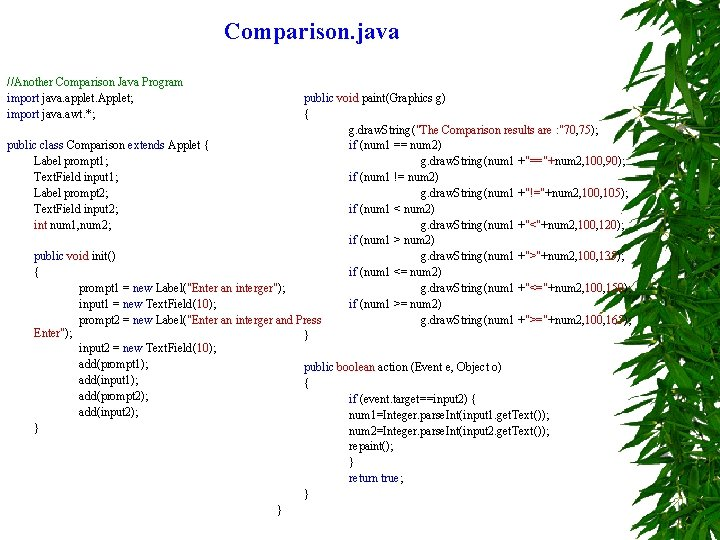Comparison. java //Another Comparison Java Program import java. applet. Applet; import java. awt. *; public void paint(Graphics g) { g. draw. String("The Comparison results are : "70, 75); public class Comparison extends Applet { if (num 1 == num 2) Label prompt 1; g. draw. String(num 1 +"=="+num 2, 100, 90); Text. Field input 1; if (num 1 != num 2) Label prompt 2; g. draw. String(num 1 +"!="+num 2, 100, 105); Text. Field input 2; if (num 1 < num 2) int num 1, num 2; g. draw. String(num 1 +"<"+num 2, 100, 120); if (num 1 > num 2) public void init() g. draw. String(num 1 +">"+num 2, 100, 135); { if (num 1 <= num 2) prompt 1 = new Label("Enter an interger"); g. draw. String(num 1 +"<="+num 2, 100, 150); input 1 = new Text. Field(10); if (num 1 >= num 2) prompt 2 = new Label("Enter an interger and Press g. draw. String(num 1 +">="+num 2, 100, 165); Enter"); } input 2 = new Text. Field(10); add(prompt 1); public boolean action (Event e, Object o) add(input 1); { add(prompt 2); if (event. target==input 2) { add(input 2); num 1=Integer. parse. Int(input 1. get. Text()); } num 2=Integer. parse. Int(input 2. get. Text()); repaint(); } return true; } }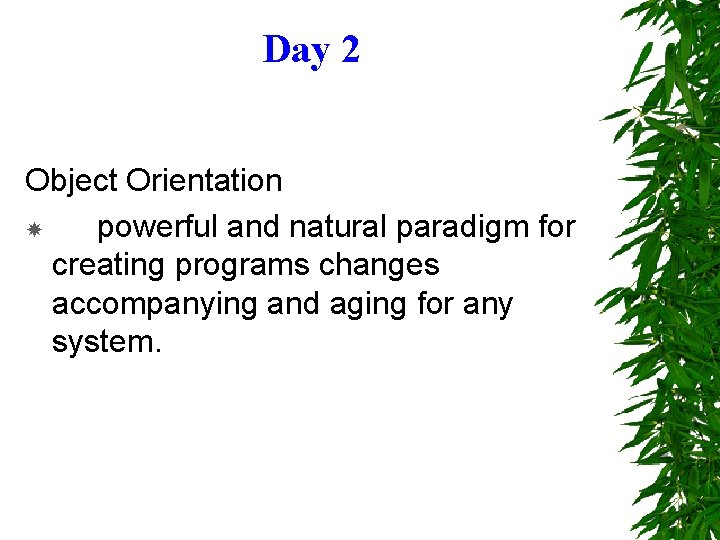Day 2 Object Orientation powerful and natural paradigm for creating programs changes accompanying and aging for any system.Common Terms Global variable is one that affects every part of the program. Pointer is actually the address of some data in memory. Passing a pointer means the address in memory is passed to subroutine. A function or subroutine is a unit of program that does a certain task and returns a value that represents the outcome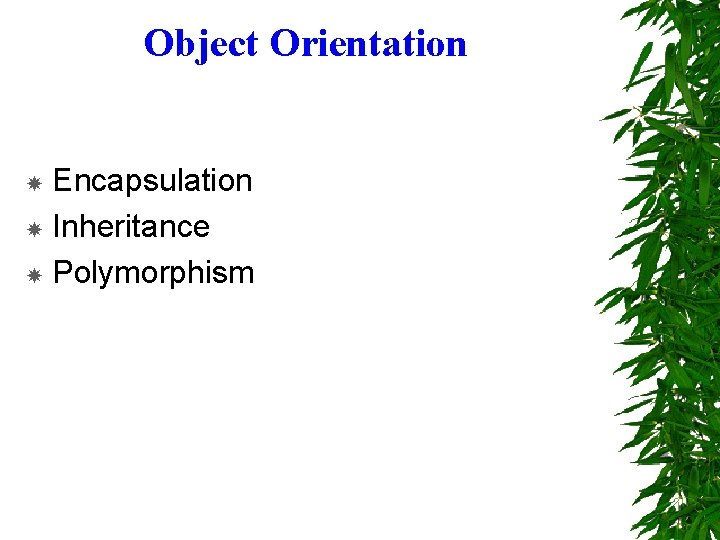Object Orientation Encapsulation Inheritance Polymorphism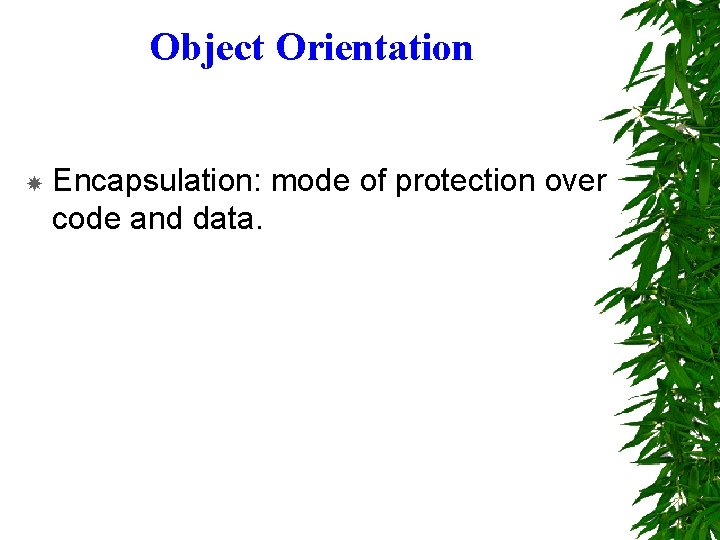Object Orientation Encapsulation: mode of protection over code and data.Common Terms A class represents an abstraction for a set of objects that share the same structure and behavior. An object is a single instance of a class that retains the structure and behavior as define by the class, sometimes objects are referred to as instance of class. A method is a message to take some action on an object. These messages are like subroutine calls or procedure language (function).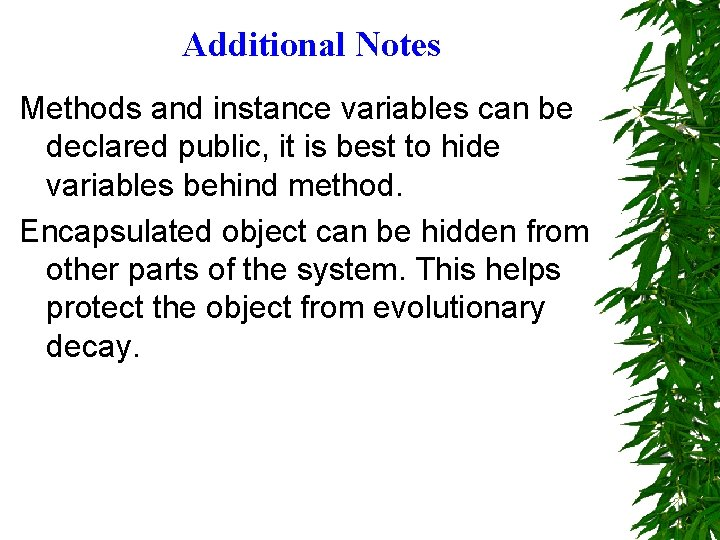Additional Notes Methods and instance variables can be declared public, it is best to hide variables behind method. Encapsulated object can be hidden from other parts of the system. This helps protect the object from evolutionary decay.A Class Vs An Instance A class is something that describes the general attributes of an object, including the types of each attributes and the methods that can operate on the object. An instance is a particular incidence of a class objects.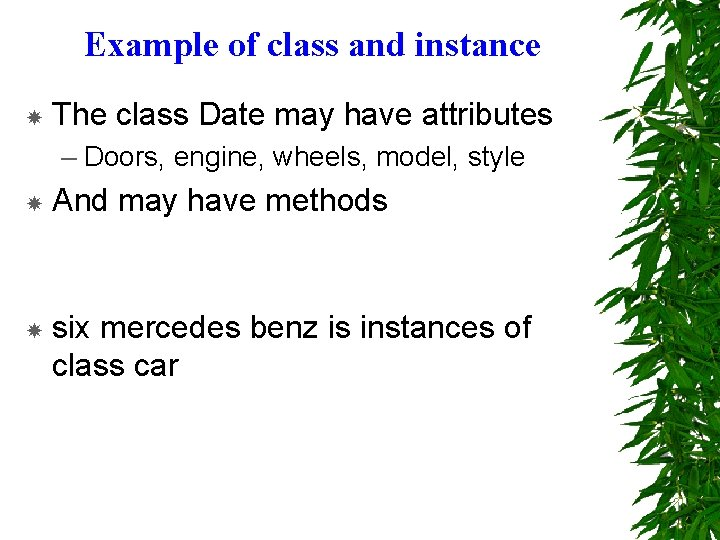Example of class and instance The class Date may have attributes – Doors, engine, wheels, model, style And may have methods six mercedes benz is instances of class car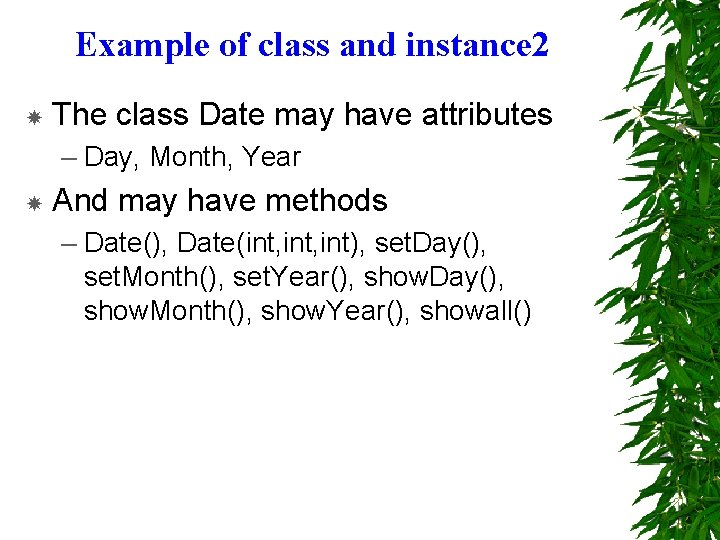Example of class and instance 2 The class Date may have attributes – Day, Month, Year And may have methods – Date(), Date(int, int), set. Day(), set. Month(), set. Year(), show. Day(), show. Month(), show. Year(), showall()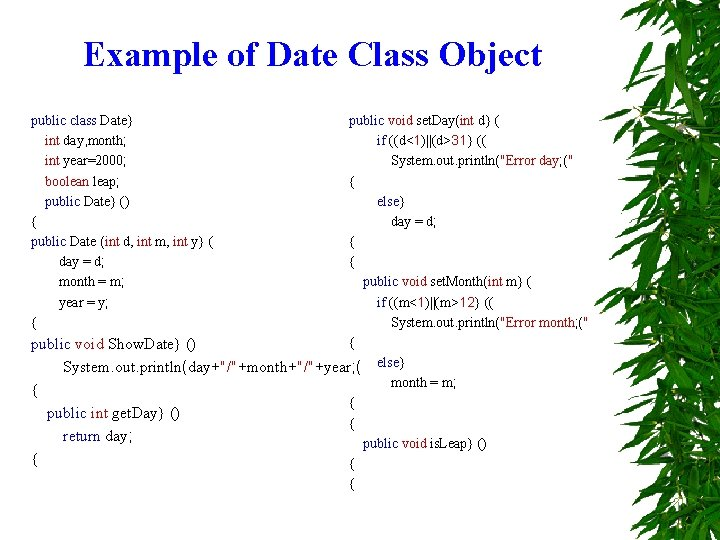Example of Date Class Object public class Date} int day, month; int year=2000; boolean leap; public Date} () { public Date (int d, int m, int y} ( day = d; month = m; year = y; { public void set. Day(int d} ( if ((d<1)||(d>31} (( System. out. println("Error day; (" { else} day = d; { { public void set. Month(int m} ( if ((m<1)||(m>12} (( System. out. println("Error month; (" { public void Show. Date} () System. out. println(day+"/"+month+"/"+year; ( else} month = m; { { public int get. Day} () { return day; public void is. Leap} () { { {Example of class Date. User (instance of Date Class) public class Date. User} public static void main (String args} ([] Date christmax = new Date; () //christmax. day=25; //christmax. month=12; //christmax. year=1999; christmax. set. Day(25; ( Date valentine = new Date(14, 2, 2000; ( christmax. Show. Date; () valentine. Show. Date; () christmax. set. Day(255; ( christmax. set. Day(30; ( christmax. set. Month(12; ( christmax. Show. Date; () { {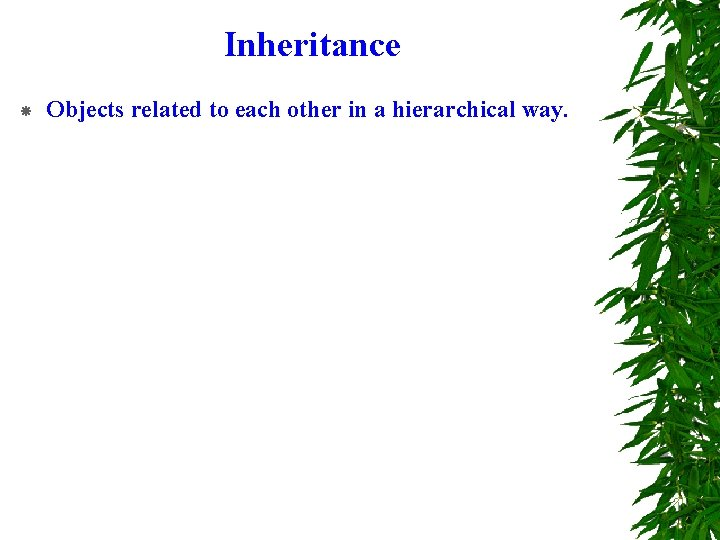Inheritance Objects related to each other in a hierarchical way.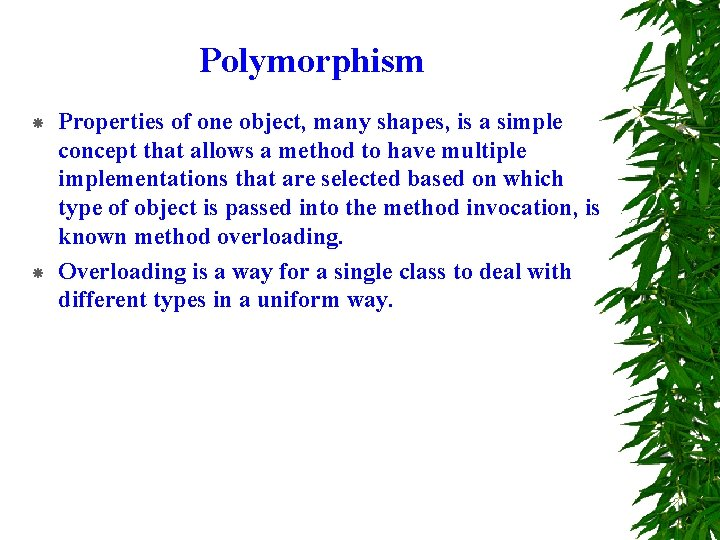Polymorphism Properties of one object, many shapes, is a simple concept that allows a method to have multiple implementations that are selected based on which type of object is passed into the method invocation, is known method overloading. Overloading is a way for a single class to deal with different types in a uniform way.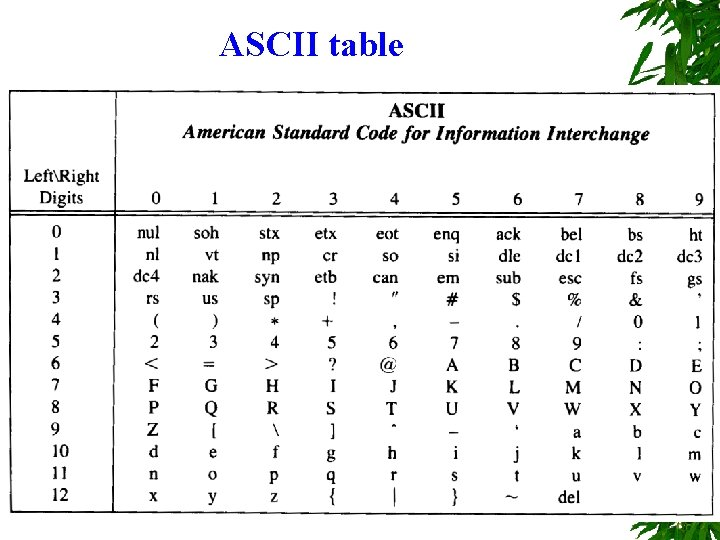ASCII tableMathematical Table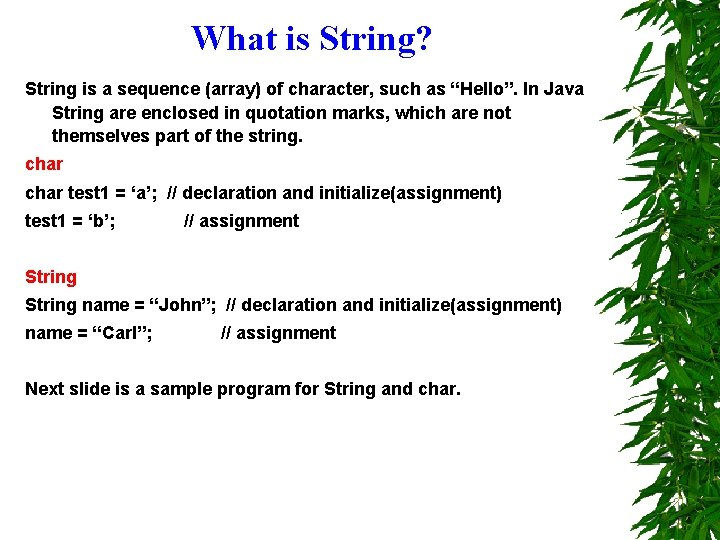What is String? String is a sequence (array) of character, such as “Hello”. In Java String are enclosed in quotation marks, which are not themselves part of the string. char test 1 = ‘a’; // declaration and initialize(assignment) test 1 = ‘b’; // assignment String name = “John”; // declaration and initialize(assignment) name = “Carl”; // assignment Next slide is a sample program for String and char.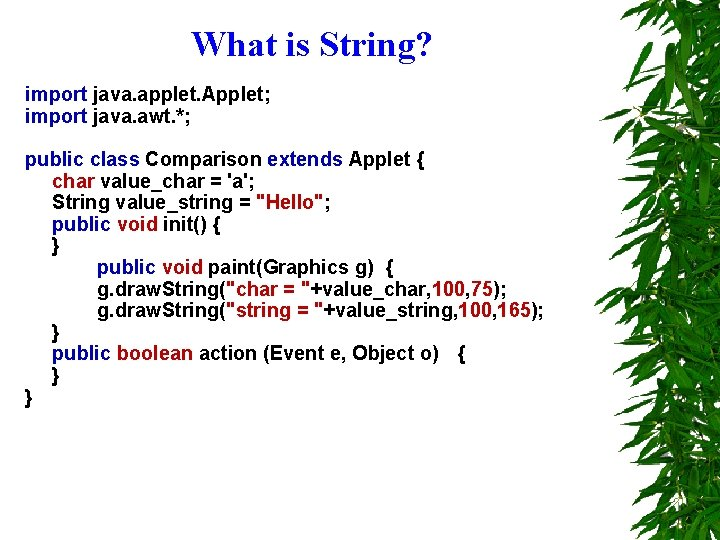What is String? import java. applet. Applet; import java. awt. *; public class Comparison extends Applet { char value_char = 'a'; String value_string = "Hello"; public void init() { } public void paint(Graphics g) { g. draw. String("char = "+value_char, 100, 75); g. draw. String("string = "+value_string, 100, 165); } public boolean action (Event e, Object o) { } }What is String? How does String kept in memory? String is a sequence of char so it keep in memory like a chain of char or we can call it array which we can do some operation with them. You can separate the string by using the substring function. Concatenation If you can separate the string, you should be able to glue it up again. String fname = “Harry”; String lname = “Hacker”; String name = fname + “ “ + lname;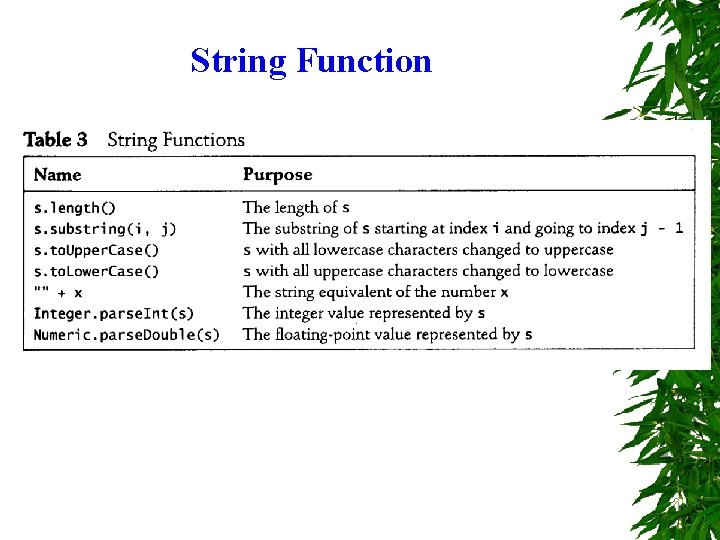String FunctionWhat is Decision is one of a tools in programming language. It help you extract your idea in logical way and tell that idea to computer. IF. format: . . if ( condition ) No Condition? { Yes statement; . . . Process }. . .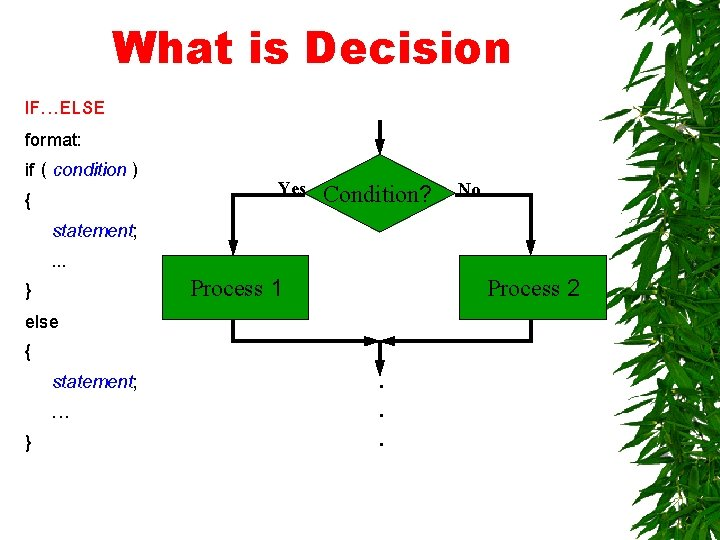What is Decision IF…ELSE format: if ( condition ) { statement; . . . } else { statement; … } Yes Condition? Process 1 No Process 2 . . .What is Decision. . Condition? IF…ELSEIF format: No if ( condition ) statement; Condition? elseif ( condition ) No statement; elseif ( condition ) Error statement; … else statement; . . Yes Process 1 Yes Process 2What is Decision. . SWITCH format: switch ( variable ) Condition? { case constant 1 : statement; No … break; Condition? case constant 2 : statement; No … break; Error default: statement … break; }. . Yes Process 1 Yes Process 2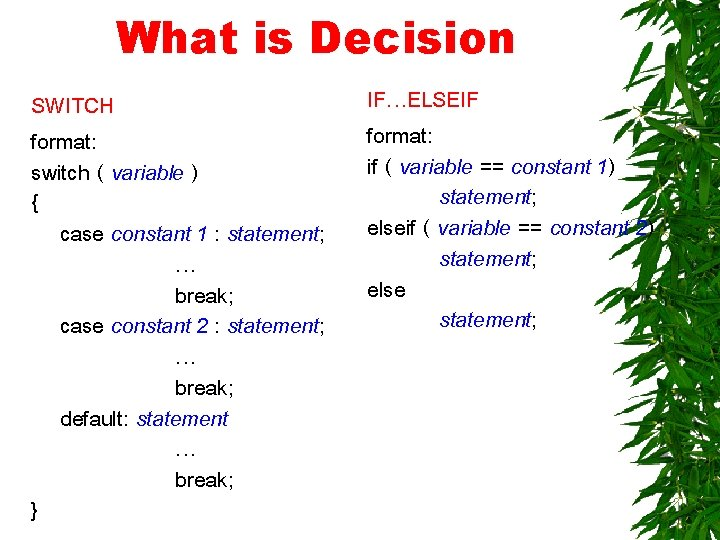What is Decision SWITCH format: switch ( variable ) { case constant 1 : statement; … break; case constant 2 : statement; … break; default: statement … break; } IF…ELSEIF format: if ( variable == constant 1) statement; elseif ( variable == constant 2) statement; else statement;Relational Operators JAVA > >= < <= == != Description Greater than or equal Less than or equal Equal Not equal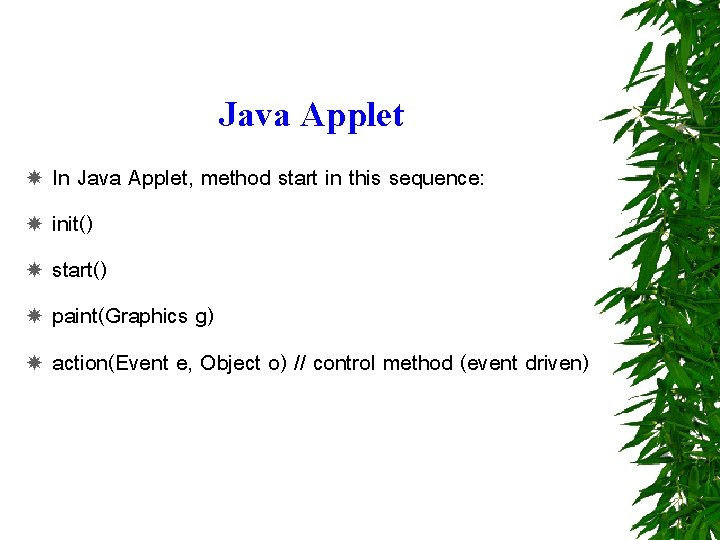Java Applet In Java Applet, method start in this sequence: init() start() paint(Graphics g) action(Event e, Object o) // control method (event driven)Hello Applet //A first Java Program import java. applet. Applet; import java. awt. Graphics; public class Hello extends Applet { public void paint (Graphics g) { g. draw. String ("Hello World", 25); } }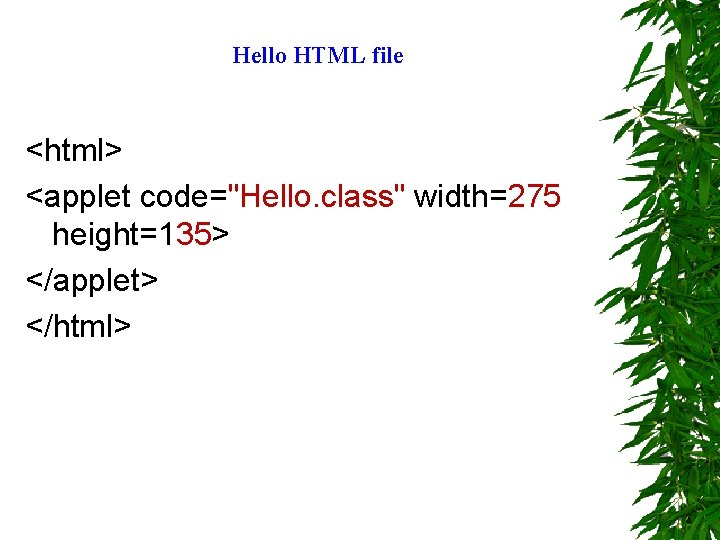Hello HTML file <html> <applet code="Hello. class" width=275 height=135> </applet> </html>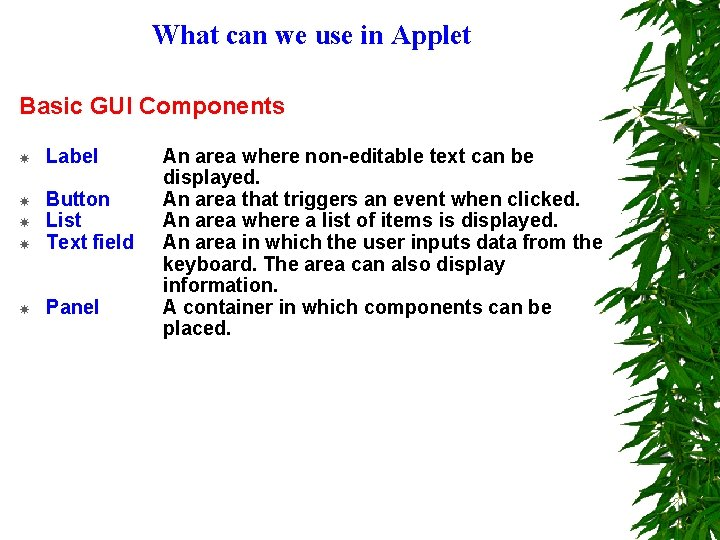What can we use in Applet Basic GUI Components Label Button List Text field Panel An area where non-editable text can be displayed. An area that triggers an event when clicked. An area where a list of items is displayed. An area in which the user inputs data from the keyboard. The area can also display information. A container in which components can be placed.What can we use in Applet Class Label constructors public Label() Constructs an empty label -- text is not displayed. Public Label ( String s ) // label text Constructs a label that displays the text s.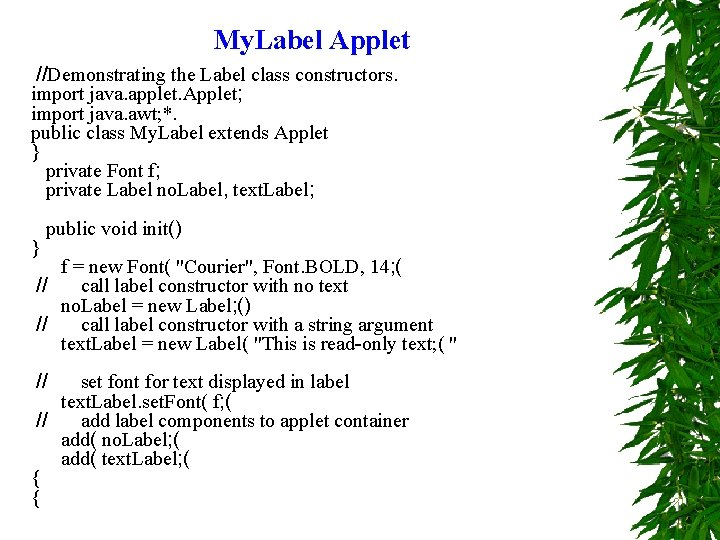My. Label Applet //Demonstrating the Label class constructors. import java. applet. Applet; import java. awt; *. public class My. Label extends Applet } private Font f; private Label no. Label, text. Label; } public void init() f = new Font( "Courier", Font. BOLD, 14; ( // call label constructor with no text no. Label = new Label; () // call label constructor with a string argument text. Label = new Label( "This is read-only text; ( " // set font for text displayed in label text. Label. set. Font( f; ( // add label components to applet container add( no. Label; ( add( text. Label; ( { {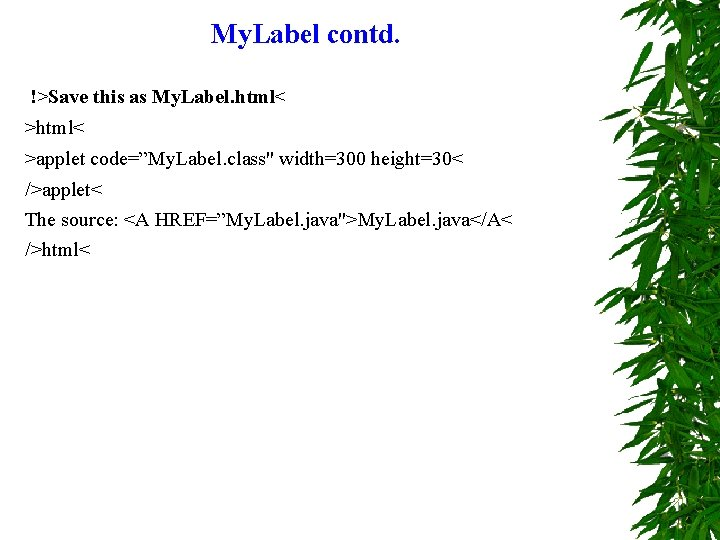My. Label contd. !>Save this as My. Label. html< >applet code=”My. Label. class" width=300 height=30< />applet< The source: <A HREF=”My. Label. java">My. Label. java</A< />html<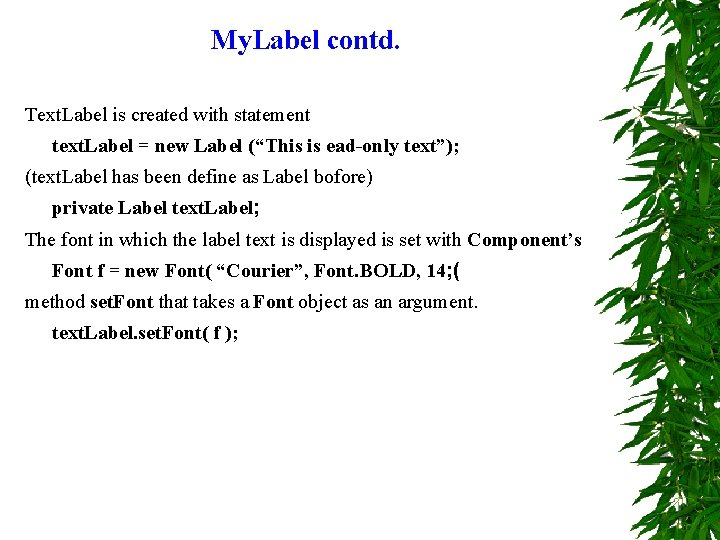My. Label contd. Text. Label is created with statement text. Label = new Label (“This is ead-only text”); (text. Label has been define as Label bofore) private Label text. Label; The font in which the label text is displayed is set with Component’s Font f = new Font( “Courier”, Font. BOLD, 14; ( method set. Font that takes a Font object as an argument. text. Label. set. Font( f );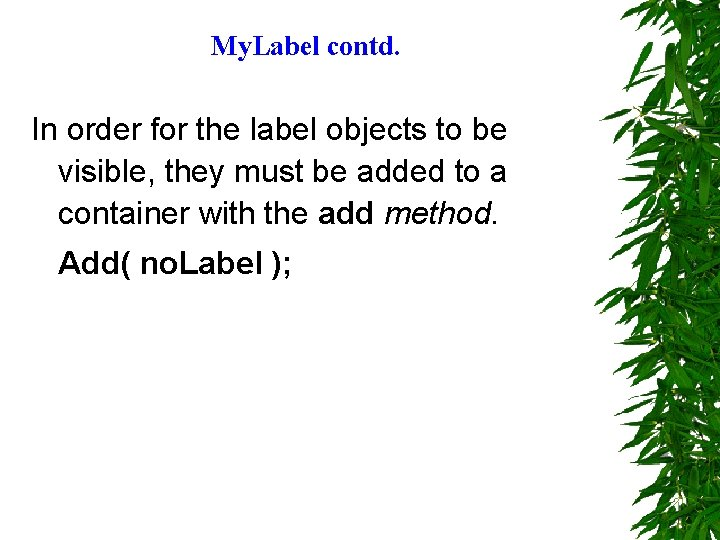My. Label contd. In order for the label objects to be visible, they must be added to a container with the add method. Add( no. Label );What can we use in Applet Method in Class Label add. Notify() Creates the peer for this label. get. Alignment() Gets the current alignment of this label. get. Text() Gets the text of this label. param. String() Returns the parameter string representing the state of this label. set. Alignment(int) Sets the alignment for this label to the specified alignment. set. Text(String) Sets the text for this label to the specified text.What can we use in Applet Class Button constructors public Button() Constructs a Button with no label Public Button ( String s ) Constructs a Button with the specified label.